• 冒泡排序 原理 和相邻的两个数值进行比较，第二个比第一个大，（他们直接就交换顺序，一直比到最后一个数） 列表：a=[1,8,7,5,3] 第一轮比较： 第二轮比较 第三轮比较： 第四轮比较 得出结论： 程序的实现 def ...
冒泡排序
原理
和相邻的两个数值进行比较，第二个比第一个大，（他们直接就交换顺序，一直比到最后一个数） 列表：a=[1,8,7,5,3] 第一轮比较：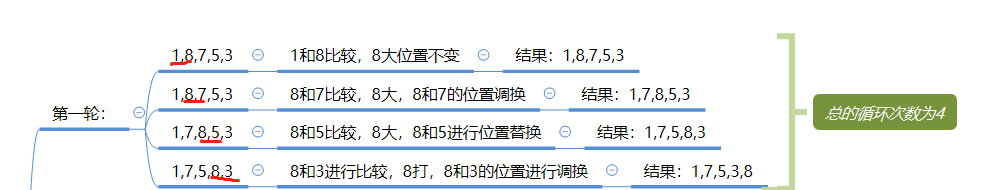第二轮比较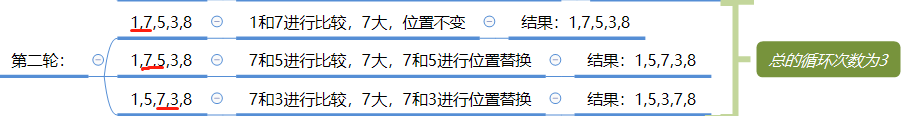第三轮比较：第四轮比较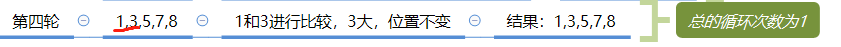得出结论：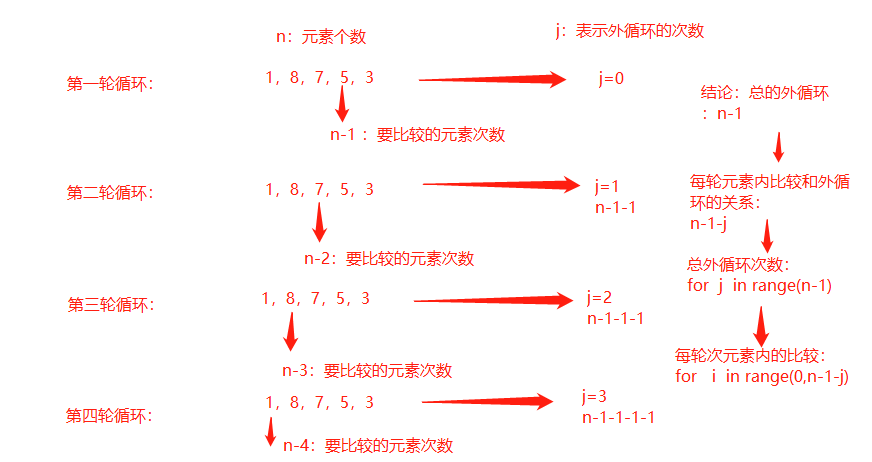程序的实现
def bubble_sort(alist):
"""冒泡排序"""

n = len(alist)         #元素个数
for j in range(n-1):   #外层轮询的次数
count = 0  #对内循环中第一次排序的时候检测，是否为顺序结构如[1,2,3,4]
for i in range(0,n-1-j):  #内存循环次数
if alist[i] > alist[i+1]:
alist[i],alist[i+1] = alist[i+1] ,alist[i]  #交换位置
count += 1  #交换了位置加1，没有则不添加

if 0 == count:   #表示数据没有交换位置，本省就是排序好的
return

时间最坏复杂读为O(n^2) :当列表之值为[3,2,1,6,5,4]
最优时间复杂读为O(n)：当列表之值为[1,2,3,4,5,6] **稳定性：**当列表里的值为[1,2,2,4,5] 里面有重复的值进行，冒泡排序的时候，位置是不交换的
展开全文python 算法 数据结构
• 面试官： 写一个冒泡排序

面试官： 写一个冒泡排序吧

冒泡排序是一个比较经典和简单的排序算法，今天我们从从算法本身，时间复杂度以及稳定性方面来看看冒泡排序，这些方面也是研究其他排序算法的一般思路
冒泡思想
在算法国内，相传有一位大师，他不喜做官，在民间传道受业，弟子三千，人称“克”
有一天，克带着得意弟子谦子去溪边游玩，看到许多大大小小的石头在溪边，克拿起了四个大小不同的石子，摆成一行，如下：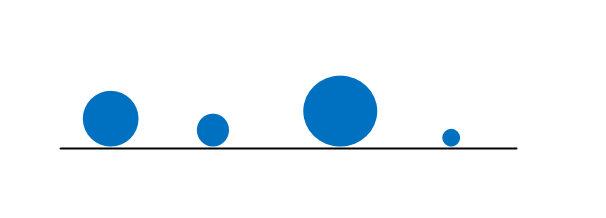问：“谦子，你如何将这些石子按照从小到大的顺序从左到右依次排列成一行？”
“先找出最大的放在右边，然后再找出次大的，放在最大的左边，按照这个规律就可以依次排好了”，谦子回答道
“那你如何找到最大的呢？”，克问道
“用肉眼看”，谦子弱弱的说了一句
“怎么可以用肉眼看，如果成千上万你也用肉眼看吗？咱们算法国以算法著称，就是让一切问题的解决都可以最终化为一个算法，可以用程序写出来”，克严厉地批评道
“那该如何找最大的呢？”，谦子问道
“你看那水
展开全文排序算法 算法
• 根据排序数组的大小来决定冒泡的次数：轮数（大小为10，则需要冒泡10次） 每一轮从最右边开始往左两两比较，如果下标大的数小于下标小的数则交换到左边，那么到达最左端时，会出现一个最小值（即每一轮都会确定下一...
核心思想：相邻的数据两两比较，如果反序则交换
时间复杂度 O(n²)

例如以下程序：
根据排序数组的大小来决定冒泡的次数：轮数（大小为10，则需要冒泡10次）每一轮从最右边开始往左两两比较，如果下标大的数小于下标小的数则交换到左边，那么到达最左端时，会出现一个最小值（即每一轮都会确定下一个数）
#include"stdio.h"

void swap1(int a[],int i,int j){
int temp = a[i];
a[i] = a[j];
a[j] = temp;
}
void swap2(int* a,int* b){
int temp = *a;
*a = *b;
*b = temp;
}
void print(int a[],int length){
for(int i=0;i<length;i++){
printf("%d ",a[i]);
}
putchar('\n');
}
void Bubble(int arr[],int length){
for(int i=0;i<length;i++){
for(int j = length -1;j>i;j--)
if(arr[j-1]>arr[j]){
swap1(arr,j-1,j);
//swap2(&arr[j-1],&arr[j]);
}
}
}

int main(){
int a = {4,1,9,5,7,2,6,8,3,10};
Bubble(a,10);
print(a,10);
return 0;
}
改进的冒泡排序（增加交换数据的标志位，避免序列已经是有序的情况下，仍在进行比较排序）
void Bubble(int arr[],int length){
int flag = 0;
for(int i=0;i<length&&flag==0;i++){
flag = 1 ;
for(int j = length -1;j>i;j--)
if(arr[j-1]>arr[j]){
flag = 0;
swap1(arr,j-1,j);
//swap2(&arr[j-1],&arr[j]);
}
}
}



展开全文• 内部排序：只用到了电脑内存而使用外存的排序方式 外部排序：同时动用了电脑内存和外存的排序方式。 插入排序 （内部排序） 在添加新的记录时，使用顺序查找的方式找到其要插入的位置，然后将新记录插入。 void ...
内部排序与外部排序 内部排序：只用到了电脑内存而不使用外存的排序方式 外部排序：同时动用了电脑内存和外存的排序方式
稳定排序与不稳定排序 通俗地讲就是能保证排序前两个相等的数其在序列的前后位置顺序和排序后它们两个的前后位置顺序相同。在简单形式化一下，如果Ai = Aj，Ai原来在位置前，排序后Ai还是要在Aj位置前。 在本篇博客中 稳定排序：插入排序， 冒泡排序， 归并排序 不稳定排序：选择排序， 快速排序
插入排序
（内部排序）
在添加新的记录时，使用顺序查找的方式找到其要插入的位置，然后将新记录插入。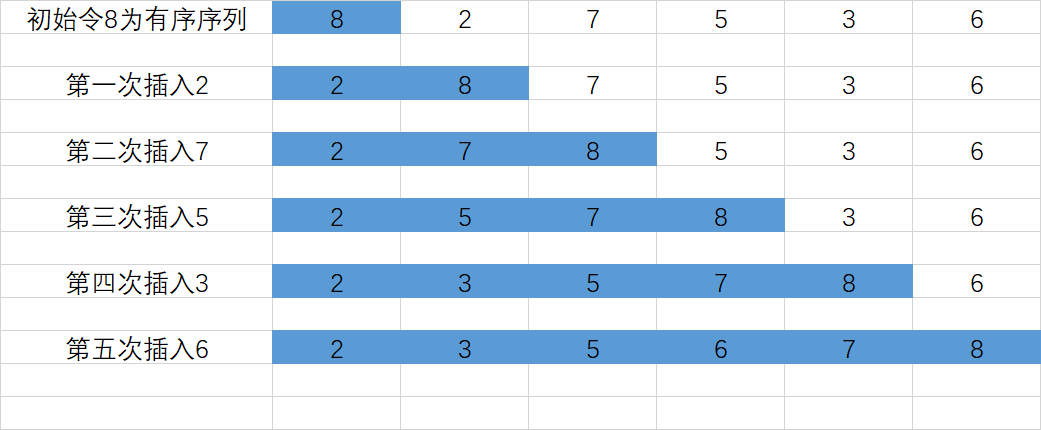void insert(int* num, int n) {
for (int i = 1; i < n; i++) {
for (int j = i; j > 0 && num[j] < num[j - 1]; j--) {
swap(num[j], num[j - 1]);
}
}
return;
}

时间复杂度为O(n^2)
冒泡排序
（内部排序） 每两两交换一次位置， 比如说第一次冒泡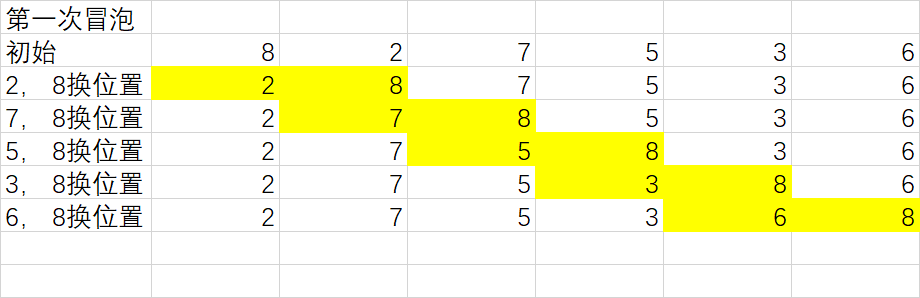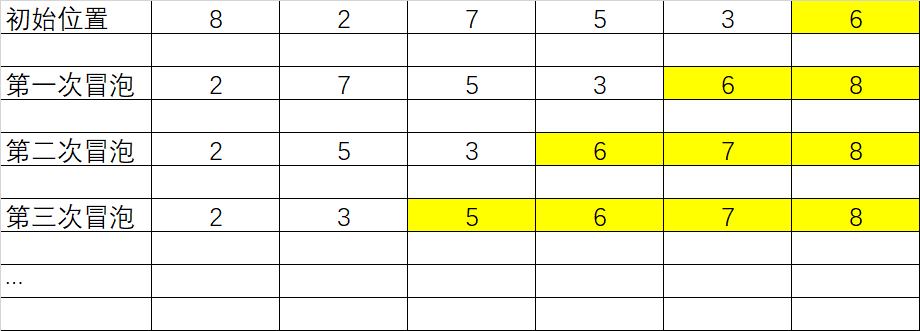时间复杂度为O(n^2)
void bubble_sort(int* num, int n) {
for (int i = 1; i < n && times; i++) {
for (int j = 0; j < n - i; j++) {
if (num[j] <= num[j + 1]) continue;
swap(num[j], num[j + 1]);
}
}
return;
}

第三轮开始可以优化
void bubble_sort(int* num, int n) {
int times = 1;
for (int i = 1; i < n && times; i++) {
times = 0;
for (int j = 0; j < n - i; j++) {
if (num[j] <= num[j + 1]) continue;
swap(num[j], num[j + 1]);
times++;
}
}
return;
}

归并排序
（外部排序） 将问题分成一些小的问题然后递归求解，将分的阶段得到的各答案合在一起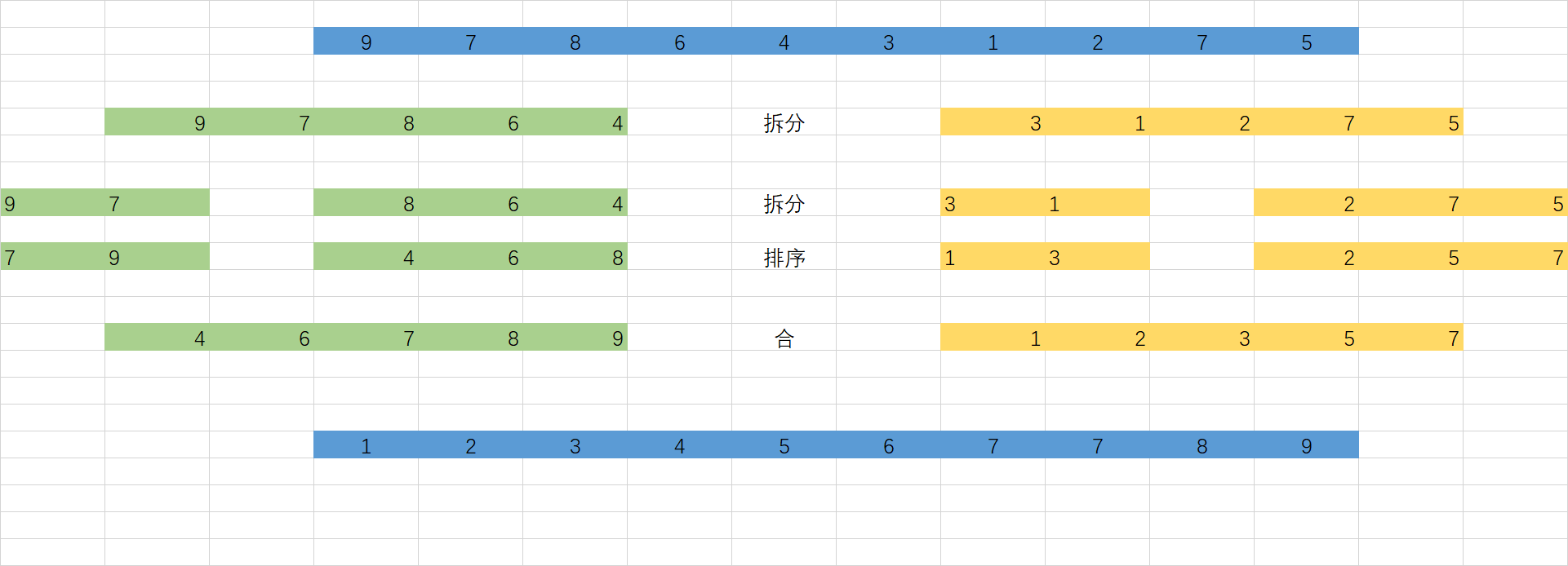void merge_sort(int* num, int l, int r) {
if (r - l <= 1) {
if (r - l == 1 && num[l] > num[r]) {
swap(num[l], num[r]);
}
return;
}
int mid = (l + r) >> 1;
merge_sort(num, l, mid);
merge_sort(num, mid + 1, r);
int* temp = (int*)malloc(sizeof(int) * (r - l + 1));
int p1 = l, p2 = mid + 1, k = 0;
while (p1 <= mid || p2 <= r) {
if (p2 > r || (p1 <= mid && num[p1] <= num[p2])) {
temp[k++] = num[p1++];
} else {
temp[k++] = num[p2++];
}
}
memcpy(num + l, temp, sizeof(int) * (r - l + 1));
free(temp);
return;
}

选择排序
(不稳定排序） 选择排序，从头至尾扫描序列，找出最小的一个元素，和第一个元素交换，接着从剩下的元素中继续这种选择和交换方式，最终得到一个有序序列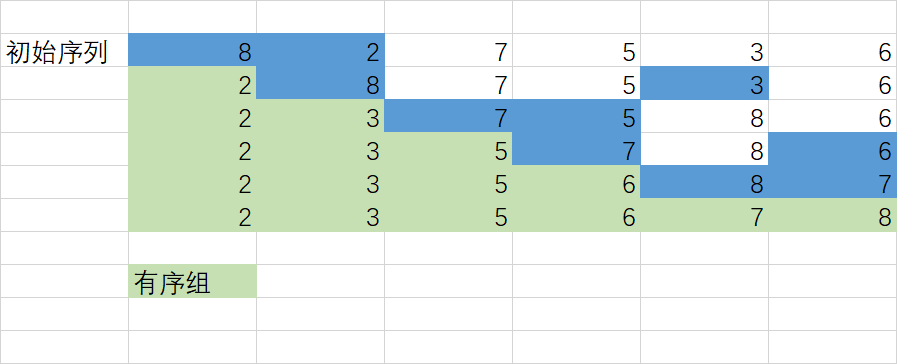void select_sort(int *num, int n) {
for (int i = 0; i < n - 1; i++) {
int ind = i;
for (int j = i + 1; j < n; j++) {
if (num[ind] > num[j]) ind = j;
}
swap(num[i], num[ind]);
}
return ;
}

时间复杂度O(n^2)
快速排序
其基本思想是随机找出一个数（通常就拿数组第一个数据就行），把它插入一个位置，使得它左边的数都比它小，它右边的数据都比它大，这样就将一个数组分成了两个子数组，然后再按照同样的方法把子数组再分成更小的子数组，直到不能分解为止。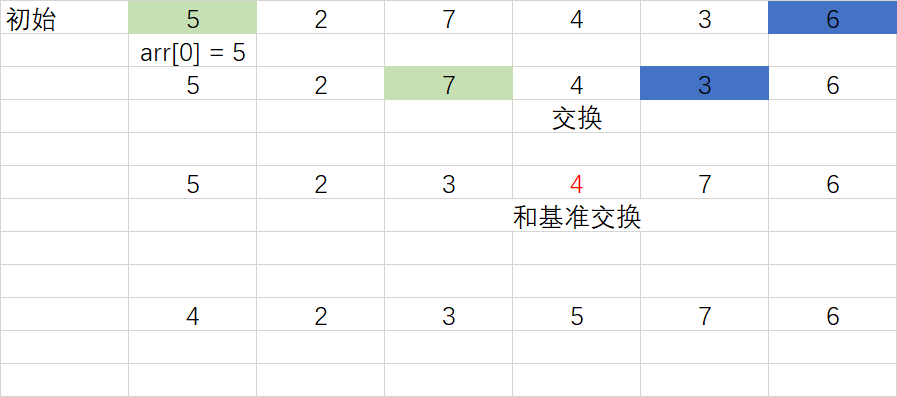//只适用于无重复数字的数组
void quick_sort(int *num, int l, int r) {
if (r < l) return;
int x = l, y = r, z = num[l];
while (x < y) {
while (x < y && num[y] >= z) y--;
if (x < y) num[x++] = num[y];
while (x < y && num[x] <= z) x++;
if (x < y) num[y--] = num[x];
}
num[x] = z;
quick_sort(num, l, x - 1);
quick_sort(num, x + 1, r);
return;
}

优化
//都可
void quick_sort(int *num, int l, int r) {
while (l < r) {
int x = l, y = r, z = num[(l + r) >> 1];
do {
while (x <= y && num[x] < z) x++;
while (x <= y && num[y] > z) y--;
if (x <= y) {
swap(num[x], num[y]);
x++, y--;
}
} while (x <= y);
quick_sort(num, x, r);
r = y;
}
return ;
}

时间复杂度O(nlogn)
展开全文• 冒泡排序是一个比较经典和简单的排序算法，今天我们从从算法本身，时间复杂度以及稳定性方面来看看冒泡排序，这些方面也是研究其他排序算法的一般思路 一、冒泡思想 在算法国内，相传有一位大师，他喜做官，在民间...算法 数据结构
• 冒泡排序是一个比较经典和简单的排序算法，今天我们从从算法本身，时间复杂度以及稳定性方面来看看冒泡排序，这些方面也是研究其他排序算法的一般思路 冒泡思想 在算法国内，相传有一位算法大师，他喜做官，一心...排序算法 算法
• 连续碰到一个关于常见排序算法稳定性判别的问题，往往还是多选，对于我以及和我一样拿不准的同学可不是一个能轻易下结论的题目，当然如果你笔试之前已经记 住了数据结构书上哪些是稳定的，哪些不是稳定的，做起来...算法 数据结构 shell
• 冒泡排序 O(n^2) O(n) O(n^2) O(1) 稳定 简单选择排序 O(n^2) O(n^2) O(n^2) O(1) 稳定 直接插入排序 O(n^2) O(n) O(n^2) O(1) 稳定 希尔排序 O(nlogn)~O(n^2) O(n^1.3) O(n^2) O...排序 快速排序 希尔排序 归并排序
• 本文来自于“涛声依旧”的投稿，微信公众号“码分享”，老刘做了部分修改。冒泡排序是一个比较经典和简单的排序算法，今天我们从从算法本身，时间复杂度以及稳定性方面来看看冒泡排序...
• 冒泡排序 原理： 在无序区间内，通过相邻数的比较，将最大的数冒泡到无序区间的最后，持续这个过程，直到数组整体有序。 图示： 实现： public static void bubbleSort(int[] array) { for (int i = 0; i < ...
• 冒泡排序，顾名思义，就是想冒泡一样，较大的泡泡或者较小的泡泡会往某个方向移动，直到所有的泡泡有序为止。 一、简单排序实现 在给出正宗的冒泡排序之前，我们来看一种简单的冒泡排序（以升序为例）。假定一定...排序算法
• 交换排序的主要算法有： （1） 冒泡排序 （2） 快速排序2、 冒泡排序（Bubble Sort）基本思想：每趟不断将相邻记录两两比较，并按“前小后大”（或“前大后小”）规则交换。步骤:它重复地走访过要排序的元素列，依次...
• 冒泡排序思路： 对于给定的n个记录，从第一个记录开始依次对相邻的两个记录进行比较，当前面的记录大于后面的记录时，交换其位置，进行一轮比较和交换位置后，n个记录中的最大记录将位于第n位；然后对前(n - 1)个...算法
• 冒泡排序是比较经典的排序方法，是一种用时间换空间的排序方法，原理： 比较相邻的元素，如果第一个比第二个大，就交换他们两个，也就说，每次比较完了，就有最大值出现。 对每一对相邻元素做同样的工作，从...
• 一、冒泡法  1、基本思想：用关键字从剩余所有元素第一个开始依次进行比较，每一趟找... 2、举例： 将arr={5，2，9，6，4，1}用冒泡法进行排序  (1)第一趟：用arr=5和其余元素依次进行比较。  5 > 2，二者位
• 稳定排序不稳定排序 这几天笔试了好几次了，连续碰到一个关于常见排序算法稳定性判别的问题，往往还是多选，对于我以及和我一样拿不准的同学可不是一个能轻易下结论的题目，当然如果你笔试之前已经记住了数据...
• 冒泡排序 package 排序算法; import java.util.Arrays; import org.junit.Test; /* * 冒泡排序： *从 arr起，前一个依次和后一个比，谁大谁放后面，直到最后一个元素，确定最大的 *从 arr起，前一个依次和...
• 005数据结构与算法Python排序与搜索排序算法的稳定冒泡排序冒泡排序的分析代码实现时间复杂度选择排序选择排序分析代码实现时间复杂度插入排序代码实现时间复杂度快速排序（工作中常用）代码实现希尔排序希尔排序...算法 数据结构 python
• 算法原理： 设待排数据元素序列的元素个数为...3. 对剩下的n-1个元素重复以上的两个动作，每趟完成后，下一趟需要排序的元素就会减少1个，直至最后的两个元素需要交换为止。 最终得到原序列从小到大排序的序列 算法排序算法 算法
• 这几天笔试了好几次了，连续碰到一个...本文是针对老是记不住这个或者想真正明白到底为什么是稳定或者不稳定的人准备的。 首先，排序算法的稳定性大家应该都知道，通俗地讲就是能保证排序前2个相等的数其在序列的...
• JS冒泡排序 原理 :依次比较相邻的两个值，如果后面的比前面的小，则将小的元素排到前面。依照这个规则进行多次并且递减的迭代，直到顺序正确。 时间复杂度，空间复杂度，稳定性 平均时间复杂度O(n*n) 最好情况...
•  由于它的简洁，冒泡排序通常被用来对于程序设计入门的学生介绍算法的概念。    冒泡排序算法的运作如下：   比较相邻的元素。如果第一个比第二个大，就交换他们两个。 对每一对相邻元素作同样的工作，从...java实现快速排序
• 1. 插入排序和冒泡排序的时间复杂度 插入排序和冒泡排序的时间复杂度相同，都是 O(n2)，在实际的软件开发里，为什么我们更倾向于使用插入排序算法而不是冒泡排序算法呢？ 2. 先看一下排序算法的几个概念 ...算法 java 数据结构
• 我只讲众多排序算法中的一小撮，也是最经典的、最常用的：冒泡排序、插入排序、选择排序、归并排序、快速排序、计数排序、基数排序、桶排序。按照时间复杂度把它们分成了三类,本文先分析冒泡、插入、选择三种排序...数据结构与算法 数组
• 比较次数和交换（或移动）次数二、排序算法的内存消耗三、排序算法的稳定性案例冒泡排序（Bubble Sort）第一，冒泡排序是原地排序算法吗？第二，冒泡排序稳定的排序算法吗？第三，冒泡排序的时间复杂度是多少？...
• 冒泡排序是我们比较常用的一种排序算法，它的原理是：从头遍历未排好序的序列，每相邻的两个元素进行比较，较大（或较小）的元素放在后面，一轮遍历之后最大（或最小）的元素已经放到最后，然后依次重复之前的步骤把...java
• 基本上冒泡排序就可以解决问题，所以熟练的掌握它很有必要。 冒泡排序  对于给定的n个记录，从第一个记录开始依次对相邻的两个记录进行比较，当前面的记录大于后面的记录时，交换其位置，进行一轮比较和交换...c语言 排序
• 冒泡排序的思想：根据序列中两个元素的比较结果来对换这两个记录在序列中的位置，将键值较大的记录向序列的尾部移动，键值较小的记录向序列的前部移动。因此，每一趟都将较小的元素移到前面，较大的元素自然就逐渐沉......